# Poincaré Sphere

### What is Poincaré Sphere?

The Poincaré sphere, shown in the figure below, is a graphical tool in real, three-dimensional space that allows convenient description of polarized light and of polarization transformations caused by propagation through devices.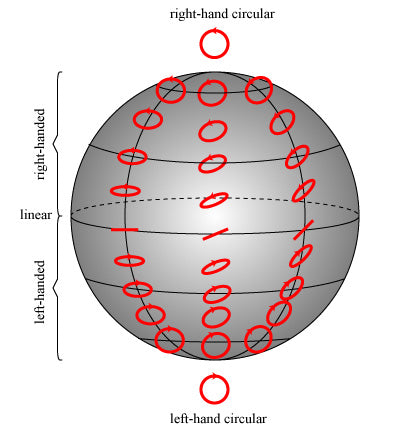Any state of polarization can be uniquely represented by a point on or within a unit sphere centered on a rectangular xyz-coordinate system as shown below.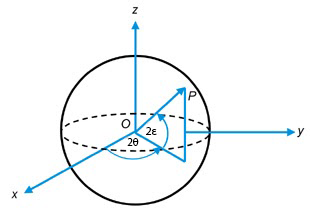Note:

Normalized Stokes parameters always have S0 = 1.

Fully polarized light (linear, circular and elliptical polarized light) is represented by a point on the surface of the Poincaré sphere. Partially polarized light, which can be considered a superposition of polarized and unpolarized light, is represented by a point within the volume of the Poincaré sphere.

• Circular states are located at the poles, with intermediate elliptical states continuously distributed between the equator and the poles.
• Right-hand elliptical states occupy the northern hemisphere, while left-hand elliptical states occupy the southern hemisphere.
• Linear states occupy the equator and only the equator.

The distance of the point from the center of the sphere O gives the degree of polarization of the light, ranging from zero at the origin (unpolarized light) to unity (1) at the sphere surface (completely polarized light).

Points close together on the sphere represent polarizations that are similar, in the sense that the interferometric contrast between two polarizations is related to the distance between the corresponding two points on the sphere.

Orthogonal polarizations with zero interferometric contrast are located diametrically opposite one another on the sphere.

The real power of the Poincaré sphere is that it enables us to determine the state of polarization of an optical beam after it has propagated through a polarizing element or several polarizing elements without carrying out the calculations.

### Derivation of Poincaré Sphere

Henri Poincaré, a famous nineteenth-century French mathematician and physicist, discovered around 1890 that the polarization ellipse (check out this article) could be represented on a complex plane

Further, he discovered that this complex plane could be projected onto a sphere in exactly the same manners as the stereographic projection as shown below.In effect, Poincaré reversed the problem of classical antiquity, which was to project a sphere onto a plane.

#### The Polarization Ellipse

Consider a Cartesian coordinate system with axes x, y, z and let the direction of propagation of a monochromatic elliptically polarized light beam be in the z direction. The equations of propagation are described bywhere Ex and Ey are the complex amplitudesAnd E0x, E0y are real quantities.

On eliminating the propagator ωt, we obtain the familiar equation of the polarization ellipse.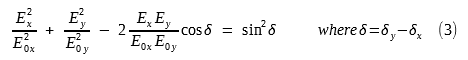Equation (3) describes an ellipse inscribed in a rectangle of sides 2E0x and 2E0y, this is shown in the figure below.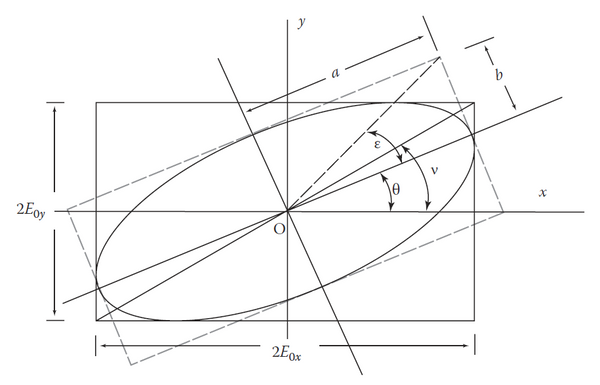The axes of the ellipse are not necessarily along the x and y axes but are rotated. The orientation of the ellipse is given by the azimuth angle θ (0 ≤ θ ≤ 180º); this is the angle between the major axis of the ellipse and the positive x axis.

The ellipticity e is the ratio of the minor axis (b) to the major axis (a) of this ellipse: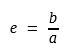The ellipticity angle ε is:The rotation angle θ and the ellipticity angle ε are the two most important parameters describing the polarization ellipse.

There are interesting relations between the parameters and angles in the polarization ellipse. They are useful for finding the coordinates of a point M on the Poincaré sphere.

We just list them below and skip the derivation process.These equations are well-known relations that appear in spherical trigonometry. It implies that the polarization ellipse on a plane can be transformed to a spherical triangle on a sphere.#### Representation of a Polarization Ellipse by a Point on a Complex Plane

The ratio between the complex amplitudes Ey and Ex defines the shape and orientation of the polarization ellipse.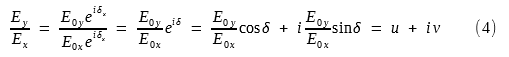The above equation utilizes Euler's formula.

Thus we get(u,v) is a point m on the complex plane as shown in the following figure.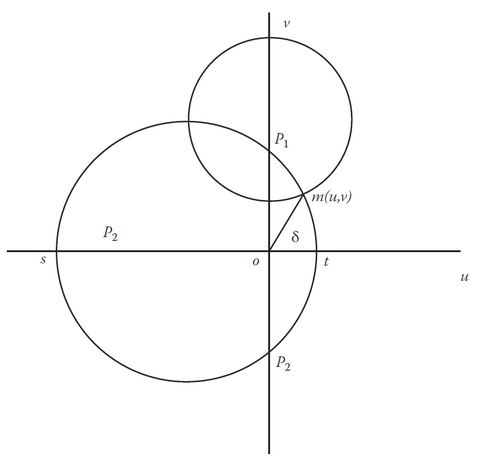Thus, the radius vector Om represents the ratio Ey/Ex, and the angle mOu represents the phase difference δ.

• The polarization is left-handed when 0 < δ < π
• The polarization is right-handed when π < δ < 2π

#### Mapping of the Complex Plane Onto a Sphere

We now consider the projection of the point m in the complex plane onto the surface of a sphere. This is shown in the following figure.The point m in the u, v plane is projected as point M on the sphere. A sphere of unit diameter (the radius r is equal to 1/2) is constructed such that point O is tangential to the u, v plane, and the points in the plane on the u axis project onto the surface of the sphere by joining them to O'.

The line OO' is the diameter, and the points p1 and p2 project onto the poles P1 and P2 of the sphere.

The point O' is called the antipode of a sphere. The vector Om projects into the arc OM of length 2v, and the spherical angle MOO' is δ.

Thus, any point M on the sphere will, as does m on the plane, represent the state of polarization of light.

#### The relation between Coordinates of M on the Sphere and Stokes Parameters

In order to find the relationship between the coordinates of point M and the parameters of the light, that is, its ellipticity angle ε, azimuth angle θ, sense (clockwise or counterclockwise), and phase difference δ, we need to express point M's coordinates (x, y, z) in terms of angles θ and ε.

This means we need to transform the coordinates of m on the u, v complex plane to M on the sphere.

Coordinates (x, y, z) of point M on the sphere

As shown in the above figure, the center of the sphere is taken as the origin of the coordinate system (x, y, z)Note: the radius of this sphere is 1/2.

Here we skip the complicated derivation steps and just list the answer. The coordinates of point M on the sphere are:Stokes Parameters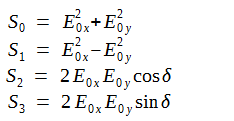Recall from the first figure (polarization ellipse), we have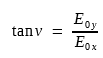We now setAnd we can put it into a right triangle relationship as shown below.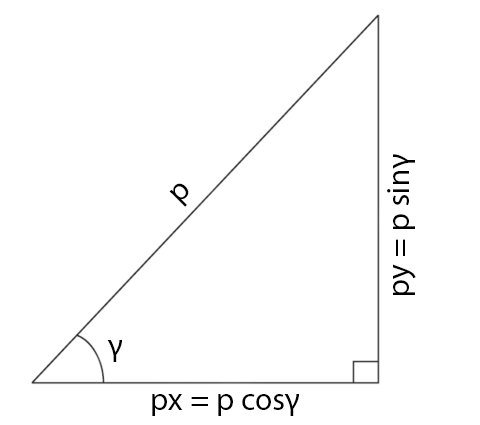Thus, we can express all Stokes parameters in terms of angle ν and δ:We can set C2 = 1/2, and then we get:The relationship

We compare (6) and (7) and see that the (x, y, z) coordinates of point M on this sphere is exactly Stokes parameters (S1, S2, S3).

Thus, the coordinates of the point M on the Poincaré sphere correspond to the Stokes parameters S1, S2, S3 of the optical beam and S0 corresponds to the radius of the sphere.

### Major Properties of the Poincaré Sphere

On the Poincaré sphere for a unit intensity (S0 = 1) we can write the Stokes parameters as (substitute angle ν in terms of azimuth angle θ and ellipticity angle ε):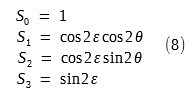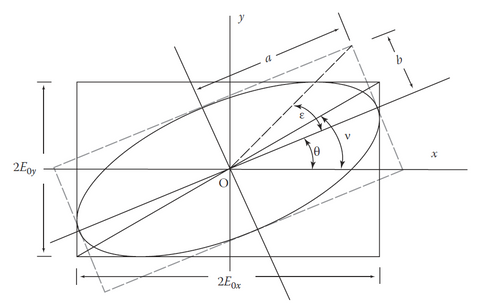#### 1. Points on the equator

When 2ε = 0, which corresponds to the equator on the Poincaré sphere, the Stokes parameters are:These equations are the Stokes parameters for linearly polarized light oriented at an angle θ.

There are four special cases:#### 2. Points on the prime meridian

When 2θ = 0, which corresponds to the prime meridian (longitude), then we get the Stokes parameters as: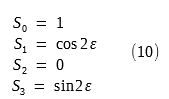There are two special cases: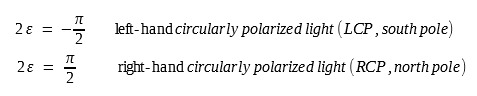### Poincaré Sphere Live Demo:

Play with Wolfram's live demo here:

http://demonstrations.wolfram.com/LightPolarizationAndStokesParameters/